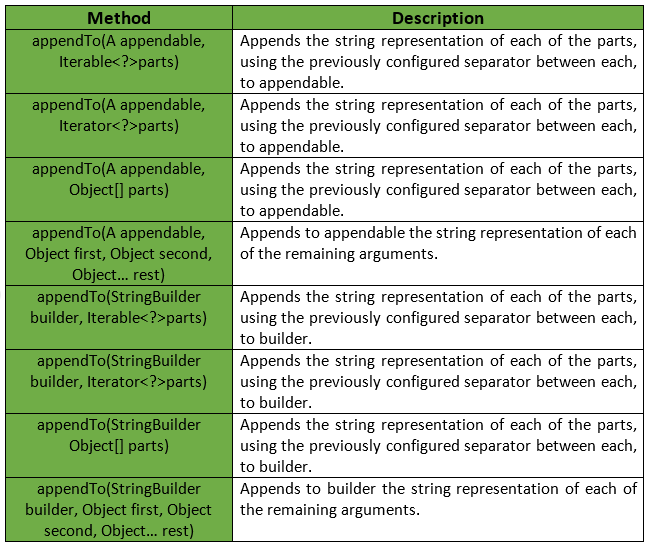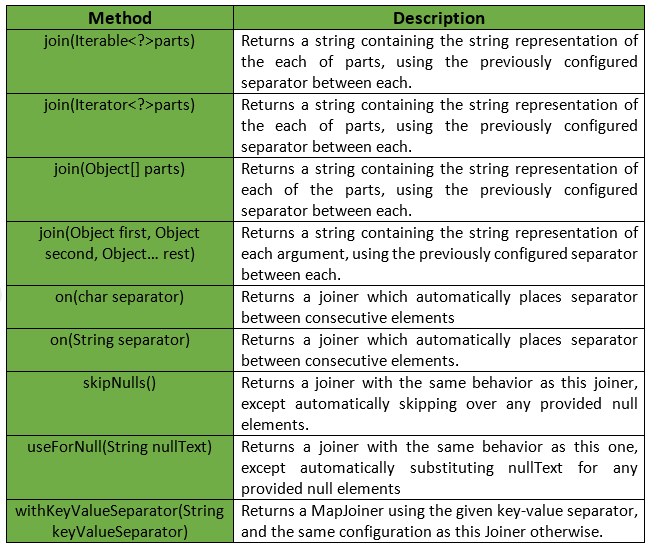Related Articles

# Joiner class | Guava | Java

• Last Updated : 15 Nov, 2018

Guava’s Joiner class provides various methods to handle joining operations on string, objects, etc. This class provides advanced functionality for the join operation.

Declaration: Following is the declaration for com.google.common.base.Joiner class :

```@GwtCompatible
public class Joiner
extends Object
```

The following table gives a brief summary of the methods provided by Guava’s Joiner class:Example:

 `// Java code to show implementation of``// Guava's Joiner class's method`` ` `import` `com.google.common.base.Joiner;``import` `java.util.*;`` ` `class` `GFG {`` ` `    ``// Driver's code``    ``public` `static` `void` `main(String[] args)``    ``{`` ` `        ``// Creating a string array``        ``String[] arr = { ``"one"``, ``"two"``, ``"three"``, ``"four"` `};``        ``System.out.println(``"Original Array: "``                           ``+ Arrays.toString(arr));`` ` `        ``// Use Joiner to combine all elements.``        ``// ... Specify delimiter in on method.`` ` `        ``// The last part of the Joiner statement, join,``        ``// can receive an Iterable (like an ArrayList) or``        ``// an Object array. It returns a String.``        ``String result = Joiner.on(``"..."``)``                            ``.join(arr);`` ` `        ``System.out.println(``"Joined String: "``                           ``+ result);``    ``}``}`
Output:
```Original Array: [one, two, three, four]
Joined String: one...two...three...four
```

Some more methods provided by Guava’s Joiner class are:Example:

 `// Java code to show implementation of``// Guava's Joiner class's method`` ` `import` `com.google.common.base.Joiner;``import` `java.util.*;`` ` `class` `GFG {`` ` `    ``// Driver's code``    ``public` `static` `void` `main(String[] args)``    ``{`` ` `        ``// Creating a string array``        ``String[] arr = { ``"one"``, ``"two"``, ``null``,``                         ``"four"``, ``null``, ``"five"` `};``        ``System.out.println(``"Original Array: "``                           ``+ Arrays.toString(arr));`` ` `        ``// Unlike the standard join method, we can``        ``// filter elements with a Joiner. With skipNulls,``        ``// null elements in an array or Iterable are removed.``        ``// Often null elements are not needed.``        ``// \$\$ Specify delimiter in on method.`` ` `        ``// The last part of the Joiner statement, join,``        ``// can receive an Iterable (like an ArrayList) or``        ``// an Object array. It returns a String.``        ``String result = Joiner.on(``'+'``)``                            ``.skipNulls()``                            ``.join(arr);`` ` `        ``System.out.println(``"Joined String: "``                           ``+ result);``    ``}``}`
Output:
```Original Array: [one, two, null, four, null, five]
Joined String: one+two+four+five
```